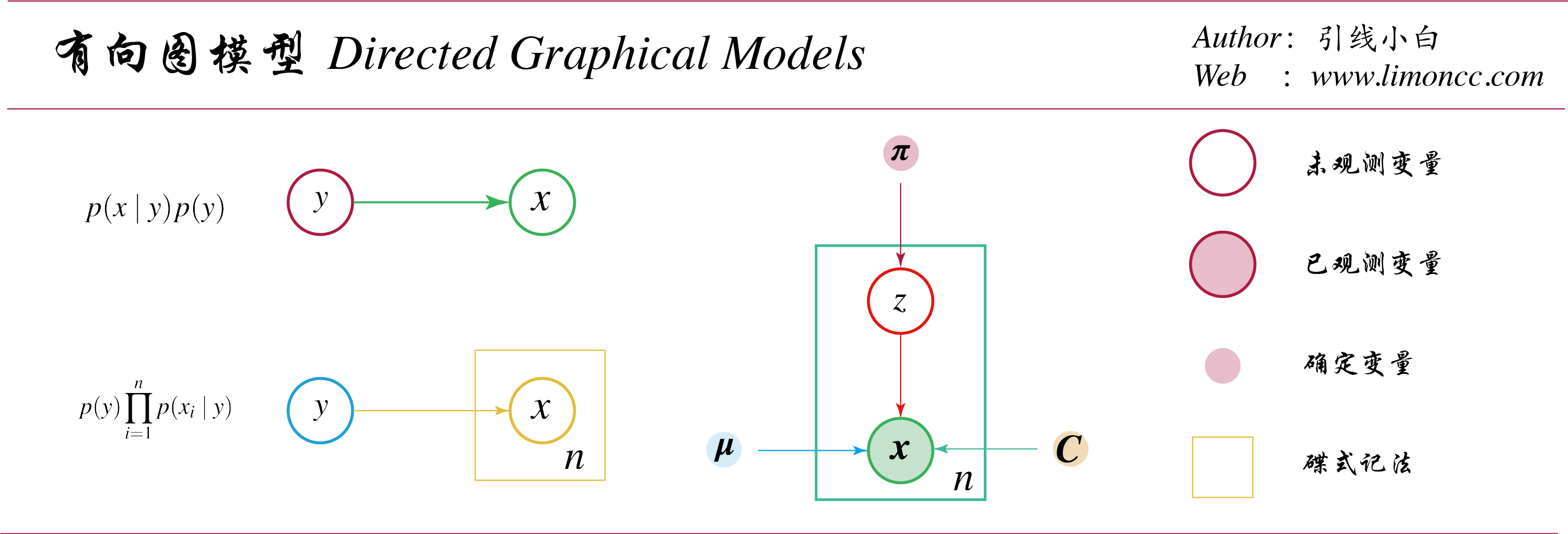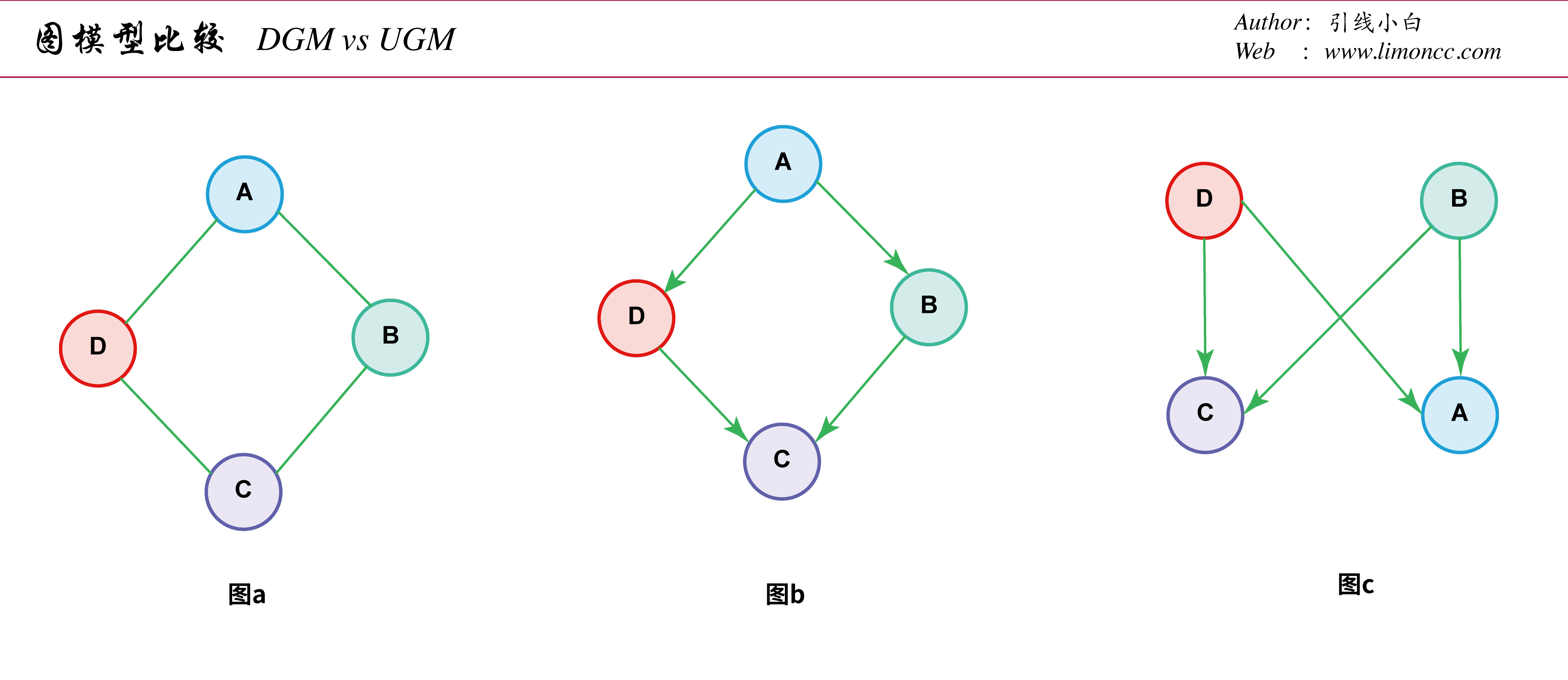Knowledge is the antidote to fear.

#### 一、问题来源

1、我们如何能简洁地表示联合分布 $\displaystyle p\big(\bm{x}\mid \bm{\theta}\big)$？
2、在合理的计算时间内，给定其他，我们如何利用这个分布来推断一组变量?
3、我们如何通过合理的数据量来学习这个分布的参数?

\begin{align} p(\bm{x})=p(x_1)\prod_{t=2}^Tp\big(x_t\mid x_{t-1}\big) \end{align}

#### 二、基本概念

##### 2.2、节点与边的符号

【节点】：引入一个符号 $\displaystyle v_s$，表示第 $\displaystyle s$个节点，这意味着我们也可以用 $\displaystyle s$表示第 $\displaystyle s$个节点。我们将会根据上下文，灵活使用这两种表示符号。

【边】：引入另外一个符号来表示边

【分类】如果一个图的所有边都是有向边，则称其为有向图 $\displaystyle \textit{(Directed Graph)}$；如果都是无向边，则称其为无向图 $\displaystyle \textit{(Undirected Graph)}$；如果皆有之，则称其为混合图$\displaystyle \textit{(Mixed Graph)}$

##### 2.6、有向图与联合分布#### 三、有向无环图模型$\displaystyle \textit{ (DGM or DAG) }$

##### 3.1、有向马尔可夫性质

【有序马尔可夫性质 $\displaystyle O$】: 给定一个有向无环图的拓扑序 $\displaystyle \{1,\cdots,k,\cdots,K\}$，则有 $\displaystyle p(\bm{x})=\prod_{k=1}^Kp\big(x_k\mid Pa(x_k)\big)$。也就是说：给定父节点，当前节点与前置节点独立
\begin{align} O=\left\{S\mid S:\forall x_k\in V(\mathcal{G})\to x_k\perp_\mathcal{G} \bm{x}_{pred(k)-pa(k)}\mid \bm{x}_{pa(k)}\right\} \end{align}

【有向局部马尔可夫性质 $\displaystyle L$】: 给定父节点，当前节点与非后代节点独立
\begin{align} L=\{S\mid S:\forall x_t\in V(\mathcal{G})\to x_t\perp_\mathcal{G} \bm{x}_{nd(t)-pa(t)}\mid \bm{x}_{pa(t)}\} \end{align}

【全局马尔可夫性质 $\displaystyle G$】
\begin{align} G=\{S \mid S:\bm{x}_A\perp_\mathcal{G}\bm{x}_B\mid \bm{x}_C\land A,B,C\subseteq V(\mathcal{G})\} \end{align}

##### 3.2、有向马尔可夫性质定理

\begin{align} O\Longleftrightarrow L \Longleftrightarrow G \end{align}

##### 3.3、有向分离与贝叶斯球算法
###### 3.3.1、迹的有向分离

1、 $\displaystyle T$ 含有链迹 $\displaystyle x\to y\to z$ 或者 $\displaystyle x\leftarrow y\leftarrow z$，且 $\displaystyle y\in E$

2、 $\displaystyle T$ 含有叉迹 $\displaystyle x \swarrow ^y \searrow z$，且 $\displaystyle y\in E$

3、$\displaystyle T$ 含有撞迹 $\displaystyle x \searrow _y \swarrow z$,且 $\displaystyle y,\notin E \land de(y)\cap E=\emptyset$

###### 3.3.2、节点集的有向分离

$\displaystyle B$有向分离，意思是说，对于每一条 $\displaystyle a\in A$到 $\displaystyle b\in B$的迹被节点集 $\displaystyle E\textit{ (containing the evidence)}$有向分离。

###### 3.3.3、有向无环图的条件独立性质

\begin{align} \bm{x}_A\perp_\mathcal{G}\bm{x}_B\mid \bm{x}_E \iff \textit{ A is d-separation from B given E} \end{align}

【链迹】考察联合分布 $\displaystyle p(x,y,z)=p(x)p(y\mid x)p(z\mid y)$，然后我们考察基于 $\displaystyle y$的条件分布：
\begin{align} p(x,z\mid y)=\frac{p(x)p(y\mid x)p(z\mid y)}{p(y)}=\frac{p(x,y)p(z\mid y)}{p(y)}=p(x\mid y)p(z\mid y)\iff x \perp z\mid y \end{align}

【叉迹】考察联合分布 $\displaystyle p(x,y,z)=p(y)p(x\mid y)p(z\mid y)$，同样我们考察 $\displaystyle y$的分布：
\begin{align} p(x,z\mid y)=\frac{p(y)p(x\mid y)p(z\mid y)}{p(y)}=p(x\mid y)p(z\mid y)\iff x \perp z\mid y \end{align}

【撞迹】考察联合分布 $\displaystyle p(x,y,z)=p(x)p(z)p(y\mid x,z)$，同样我们考察 $\displaystyle y$的分布：
\begin{align} p(x,z\mid y)=\frac{p(x)p(z)p(y\mid x,z)}{p(y)}\iff x \require{cancel} \cancel{\perp} z\mid y \end{align}

\begin{align} p(x,z)=\int p(x)p(z)p(y\mid x,z) dy=p(x)p(z)\iff x\perp z \end{align}

###### 3.3.4、贝叶斯球算法##### 3.4、马尔可夫毯与全条件

\begin{align} mb(t)=ch(t)\cup pa(t) \cup copa(t) \end{align}

\begin{align} p\big(x_t\mid \bm{x}_{-t}\big)= \frac{ p\big(x_t ,\bm{x}_{-t}\big)}{ p\big( \bm{x}_{-t}\big)} \end{align}

\begin{align} p\big(x_t\mid \bm{x}_{-t}\big) \propto p \big(x_t\mid \bm{x}_{pa(t)}\big)\prod_{s\in ch(t)}p \big(x_s\mid \bm{x}_{pa(s)}\big) \end{align}

#### 四、图模型与联合概率分布

##### 4.1、图模型的条件独立性质与 $\displaystyle \text{I-Map}$

\begin{align} \bm{x}_A\perp_\mathcal{G}\bm{x}_B\mid \bm{x}_C \end{align}

\begin{align} \mathcal{I}(\mathcal{G})\subseteq \mathcal{I}(p) \end{align}

##### 4.2、因子分解

$。如果$ \mathcal{I}(\mathcal{G})= \mathcal{I}(p)$，那么就称$ \mathcal{G}$为$ p$的完美映射 (Perfect Map)$ \text{P-Map}$，换句话说，图可以表示分布的所有(且唯一)条件独立性质。问题就变为：对于不同分布集合，是有向图模型还是无向图模型是完美映射。从这个意义上说，两者都不是比另一个更强大的表示语言。 一些条件独立关系的例子可以完全由有向图模型建模，而无向图模型不能。考虑一个$ v$结构$ A \searrow _C \swarrow B$，这表示$ A\perp B$且$\require{cancel} A\cancel{\perp} B\mid C$。如果我们去掉箭头，得到$ A-C-B$，这表示$\require{cancel} A\cancel{\perp} B$且$ A\perp B\mid C$。这种去掉箭头的操作显然是不对的。事实上，没有无向图模型能精准完全唯一地表达由$ v$结构编码的两个条件独立性质。一般的，无向图模型的条件独立性质是单调的，这里的单调有如下含义：如果$ A \perp B \mid C$，则$ A\perp B \mid \big(C\cup D\big)$。但在有向图模型中，条件独立性质是非单调的，因为存在附加变量的条件作用可以消除由于解释消除$\displaystyle \textit{(explaining away)} $生产的条件独立性质。图模型比较 一些条件独立关系的例子可以完全由无向图模型建模的，而有向图模型不能。可以考虑如图(a)所示的$ 4$环。图(b)显示了用有向图模型建模的尝试，它能正确表达$ A\perp C \mid B,D$，但表达的$ B\perp D\mid A$是错误的。图(c)是另一个不正确的有向图模型，它正确地编码了$ A\perp C \mid B,D$，但是编码的$ B\perp D\$是错误的。事实上，没有有向图模型能够精准完全唯一地表达由这个由无向图模型编码的条件独立陈述集合。

#### 七、总结

1、概率是一个好工具，这个工具的核心在于概率的条件独立性质。或者说高级一点条件期望。没有条件期望，概率论不过是测度论的一个表现形式。正是我们定义了条件概率，概率论才枝深叶茂起来。但是表达条件概率不仅仅用数学公式，还可以用图。于是一门新的学科诞生了：概率图。
2、无论是无向图、有向图，其核心都是一组条件概率假设(或者条件概率陈述)。而在非常宽泛条件下，一组条件概率假设和一个图表意是一致。这就奠定了概率图应用的基础。
3、有了图模型这一强有力的可视化建模工具，我们足矣为各种复杂场景建模，星辰大海就在前方，我们将继续探索。

 版权声明由引线小白创作并维护的柠檬CC博客采用署名-非商业-禁止演绎4.0国际许可证。本文首发于柠檬CC [ https://www.limoncc.com ] , 版权所有、侵权必究。 本文永久链接 https://www.limoncc.com/机器学习/2017-03-10-概率图基础/

'